# Perimeter: Grid squares and polygons

### Perimeter: Grid squares and polygons

In this lesson, we will learn:

• Perimeter is the straight-line measurement of the total distance to trace around a shape
• The perimeter of a polygon can be found by adding the lengths of all sides together
• The perimeter of a figure made of grid squares is found by tracing around the outside edges only
• The formula shortcuts for the perimeter of rectangular figures made of grid squares

Notes:

• Perimeter is the measurement around the outside of a two-dimensional shape
• For a polygon (shape with straight sides only), the perimeter can be calculated by adding all the sides in the shape together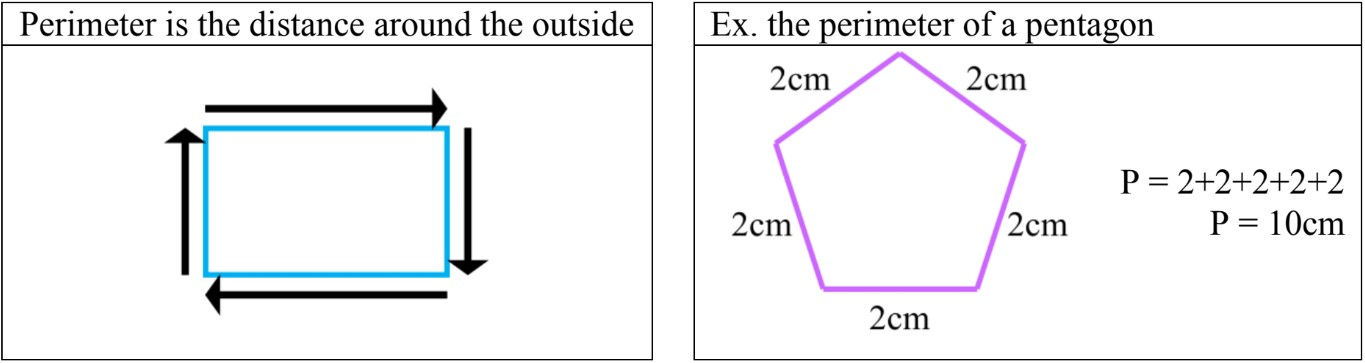• Grid squares are building blocks of figures and measure 1cm on all sides.
• To find the perimeter of a shape made of grid squares, count each edge around the OUTSIDE of the shape only.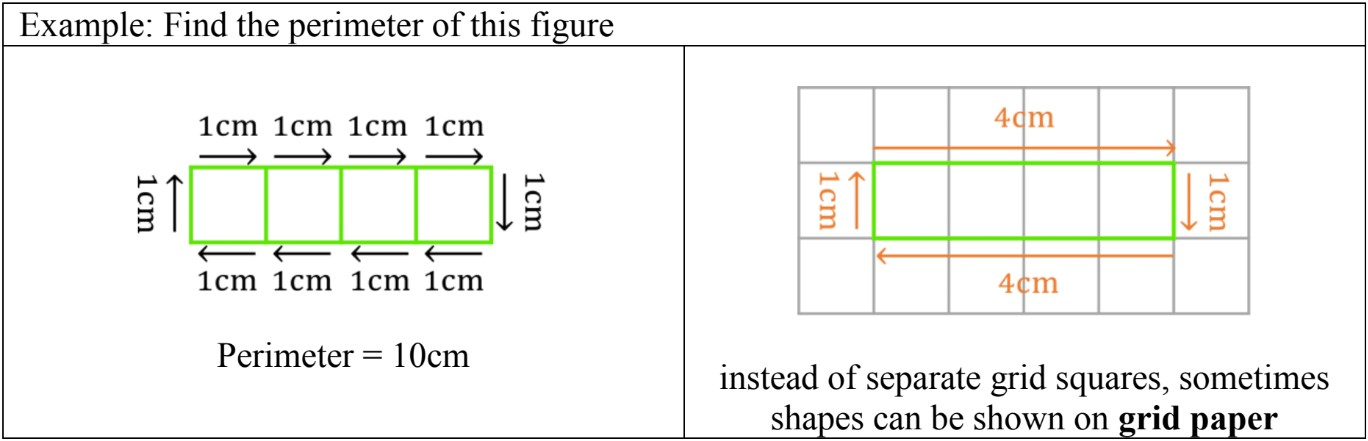• If grid squares make a rectangular figure (4-sided polygon with 2 pairs of matching sides), then a short cut can be used for finding the perimeter:
• Perimeter =2 × length + (2 × width)
• OR Perimeter =2 × (length + width)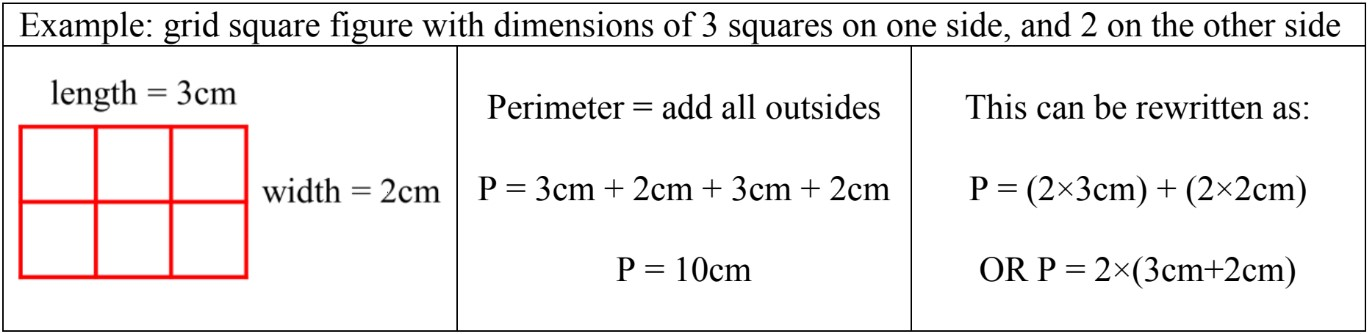#### Lessons

• Introduction
Introduction to Perimeter - Grid Squares and Polygons:
a)
What is perimeter?

b)
How to find the perimeter of polygons

c)
How to find perimeter of figures with grid squares

• 1.
Perimeter of polygons
Calculate the perimeter of the polygon. Remember to write the units in your answer.
a)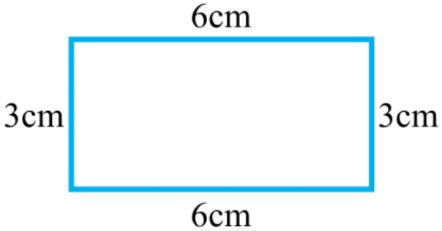b)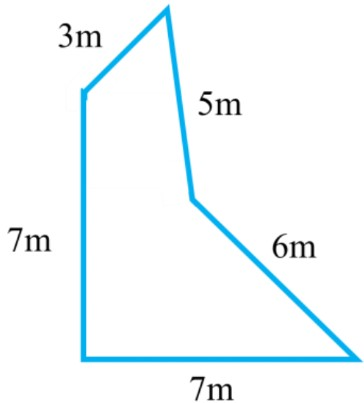c)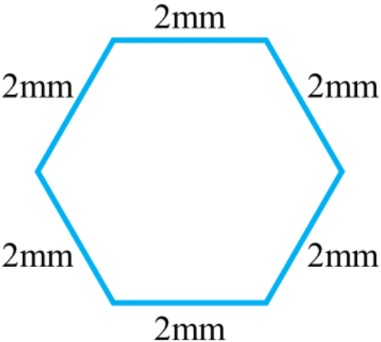• 2.
Perimeter by tracing the outside of grid squares
Trace around the grid squares to find the perimeter (in centimeters)
a)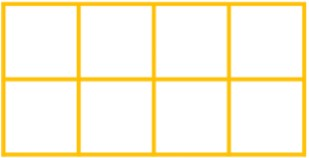b)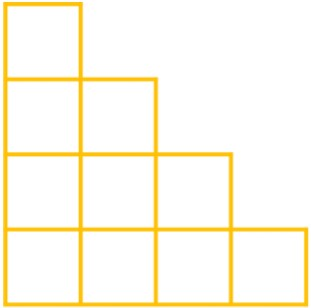c)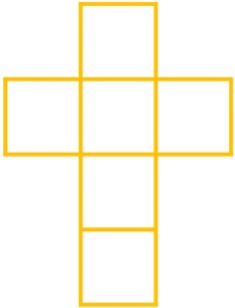• 3.
Perimeter equations with addition or multiplication
Fill in the blanks for the rectangle made of grid squares. Complete the equations for the perimeter.
a)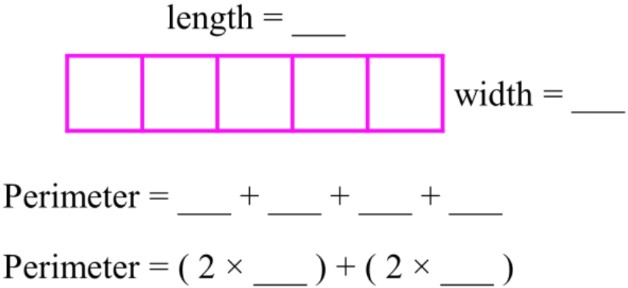b)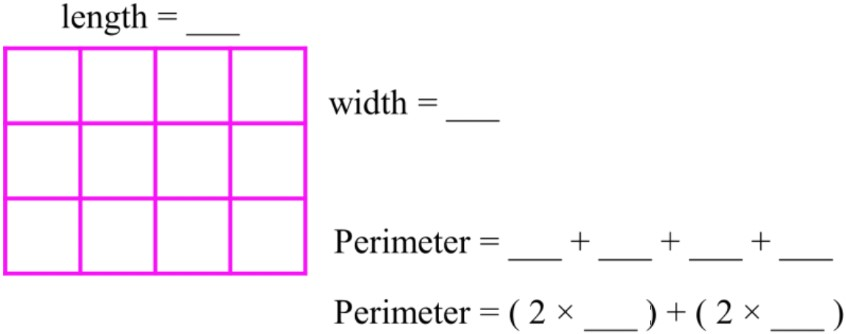c)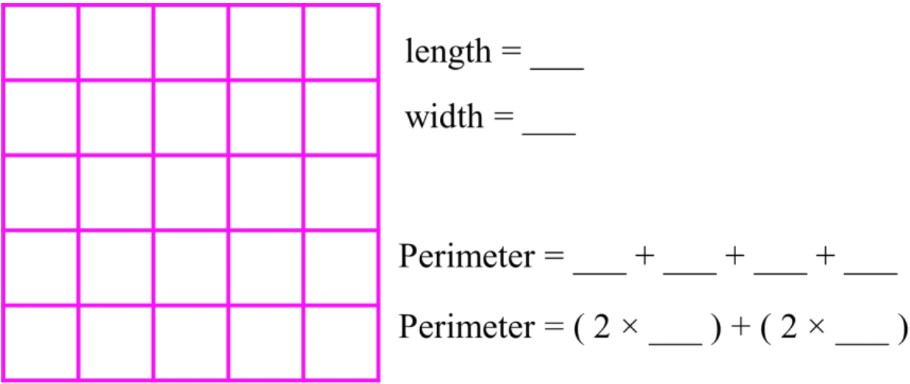• 4.
Perimeter of shapes on grid paper
A centimeter grid paper is shown with a figure drawn on it.
a)
What is the perimeter of the figure?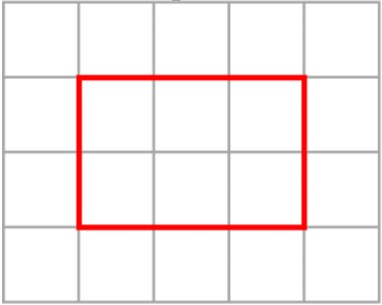b)
Different shapes can have the same perimeter. Draw another rectangle with the same perimeter.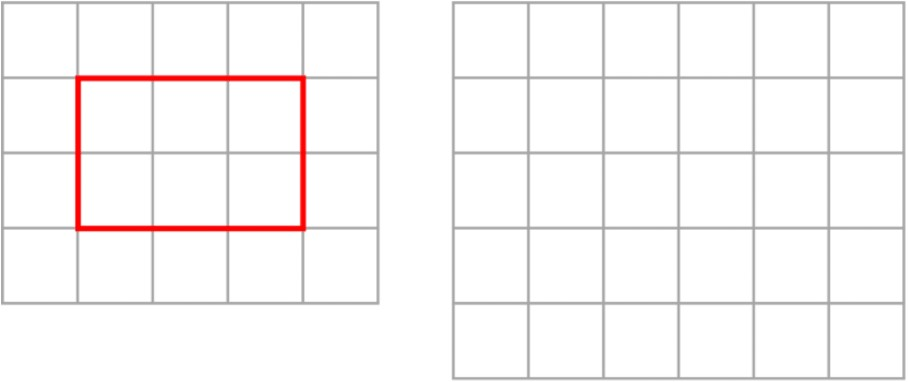c)
Two figures with the same perimeter are shown below. Are they made out of the same number of grid squares? Explain why or why not.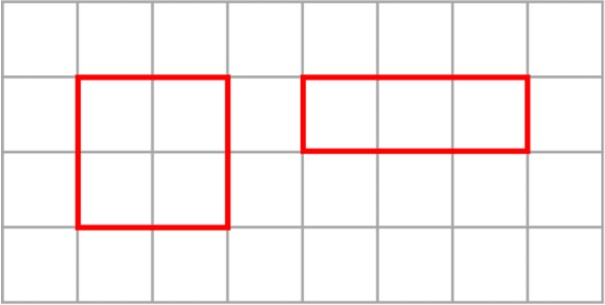• 5.
Perimeter of polygons - word problems
Use your knowledge of polygons to calculate the perimeter.
a)
What is the perimeter of a regular hexagon with 5cm on each side?

b)
What is the perimeter of a rectangle with a length of 4m and a width of 9m?

c)
If a regular octagon has a perimeter of 56mm, what is the length of each side?